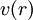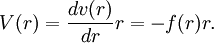Difference between revisions of "Virial (disambiguation)"

The virial of a central interaction potential$v(r)$ is defined by$V(r)= \frac{dv(r)}{dr} r = - f(r) r .$